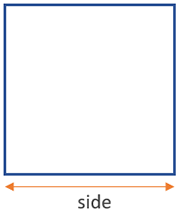# Perimeter of a Square Calculator

Use this calculator to easily calculate the perimeter of a square, given its side in any metric: mm, cm, meters, km, inches, feet, yards, miles...

### Calculation results

12 cm Square perimeter
Share calculator:

Embed this tool:
get code

## Perimeter of a square formula

The formula is: side x 4 and the result will be in whatever metric you did the measurement in: mm, cm, dm, meters or in, ft, yards, etc. This is the formula used in our perimeter of a square online calculator.

## How to calculate the perimeter of a square?

The perimeter of a square is one of the easiest to calculate in that it requires just one measurement of the square to be known - its side:From this one measurement, one just needs to multiply it by 4 to get to the perimeter, so the formula used in this perimeter of a square calculator is quite simple. The simplicity of the square is why it is usually one of the first figures geometry students get familiarized with, but in reality, we rarely deal with square objects. Square geometry is one way to understand the arithmetic square operation (raising a number to the 2-nd power), as well as square roots.

Another way to think about the square is as a special case of a rhombus - a geometrical figure with equal sides and opposite equal angles, a kite (two pairs of adjacent equal angles), a trapezoid (one pair of opposite sides are parallel), a parallelogram (all opposite sides are parallel), and, of course, a rectangle, in which opposite sides are equal and has only right angles. Thus, a square has all of the properties of these shapes, combined - diagonals bisect at 90°, diagonals bisect the square angles, diagonals are equal, the sides are equal, opposite sides are equal, all angles are equal (90°). The square is symmetrical in many ways.

## Example: find the perimeter of a square

To apply the formula, assume the side of a square is given to be 2 inches. Then the perimeter is simply 2 x 4 = 8 inches. For a real-world example, take a square pool that you need to encompass in rope completely. One side measures 10 meters. To find the perimeter of the square pool just multiply 10 by 4 to get 40 meters.

#### Cite this calculator & page

If you'd like to cite this online calculator resource and information as provided on the page, you can use the following citation:
Georgiev G.Z., "Perimeter of a Square Calculator", [online] Available at: https://www.gigacalculator.com/calculators/perimeter-of-square-calculator.php URL [Accessed Date: 27 Mar, 2023].